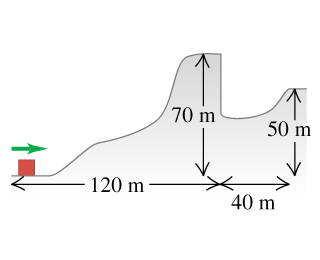# Problem: A 2.8-kg block slides over the smooth, icy hill shown in the figure. The top of the hill is horizontal and 70 m higher than its base.What minimum speed must the block have at the base of the 70-m hill to pass over the pit at the far (righthand) side of that hill?

###### FREE Expert Solution

We'll use the kinematic equation:

$\overline{){\mathbf{∆}}{\mathbf{y}}{\mathbf{=}}{{\mathbf{v}}}_{{\mathbf{0}}}{\mathbf{t}}{\mathbf{-}}\frac{\mathbf{1}}{\mathbf{2}}{\mathbf{g}}{{\mathbf{t}}}^{{\mathbf{2}}}}$

Consider the conservation of energy:

$\overline{){\mathbf{∆}}{\mathbf{U}}{\mathbf{=}}{\mathbf{∆}}{\mathbf{K}}}$

The block is starting from rest, so v= 0

80% (336 ratings)###### Problem Details

A 2.8-kg block slides over the smooth, icy hill shown in the figure.The top of the hill is horizontal and 70 m higher than its base.

What minimum speed must the block have at the base of the 70-m hill to pass over the pit at the far (righthand) side of that hill?

Frequently Asked Questions

What scientific concept do you need to know in order to solve this problem?

Our tutors have indicated that to solve this problem you will need to apply the Solving Projectile Motion Using Energy concept. You can view video lessons to learn Solving Projectile Motion Using Energy. Or if you need more Solving Projectile Motion Using Energy practice, you can also practice Solving Projectile Motion Using Energy practice problems.

What professor is this problem relevant for?

Based on our data, we think this problem is relevant for Professor Crosson's class at UNM.